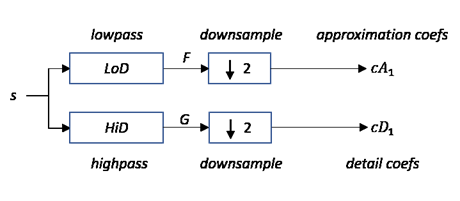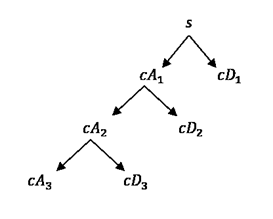# wavedec

1-D wavelet decomposition

## Syntax

``[c,l] = wavedec(x,n,wname)``
``[c,l] = wavedec(x,n,LoD,HiD)``

## Description

example

````[c,l] = wavedec(x,n,wname)` returns the wavelet decomposition of the 1-D signal `x` at level `n` using the wavelet `wname`. The output decomposition structure consists of the wavelet decomposition vector `c` and the bookkeeping vector `l`, which contains the number of coefficients by level. The structure is organized as in this level-3 decomposition diagram.```
````[c,l] = wavedec(x,n,LoD,HiD)` returns the wavelet decomposition using the specified lowpass and highpass wavelet decomposition filters `LoD` and `HiD`, respectively.```

## Examples

collapse all

Load and plot a one-dimensional signal.

```load sumsin plot(sumsin) title('Signal')```Perform a 3-level wavelet decomposition of the signal using the order 2 Daubechies wavelet. Extract the coarse scale approximation coefficients and the detail coefficients from the decomposition.

```[c,l] = wavedec(sumsin,3,'db2'); approx = appcoef(c,l,'db2'); [cd1,cd2,cd3] = detcoef(c,l,[1 2 3]);```

Plot the coefficients.

```subplot(4,1,1) plot(approx) title('Approximation Coefficients') subplot(4,1,2) plot(cd3) title('Level 3 Detail Coefficients') subplot(4,1,3) plot(cd2) title('Level 2 Detail Coefficients') subplot(4,1,4) plot(cd1) title('Level 1 Detail Coefficients')```## Input Arguments

collapse all

Input signal, specified as a real-valued vector.

Data Types: `double`

Level of decomposition, specified as a positive integer. `wavedec` does not enforce a maximum level restriction. Use `wmaxlev` to ensure that the wavelet coefficients are free from boundary effects. If boundary effects are not a concern in your application, a good rule is to set `n` less than or equal to `fix(log2(length(x)))`.

Data Types: `double`

Analyzing wavelet, specified as a character vector or string scalar.

### Note

`wavedec` supports only Type 1 (orthogonal) or Type 2 (biorthogonal) wavelets. See `wfilters` for a list of orthogonal and biorthogonal wavelets.

Wavelet decomposition filters, specified as a pair of even-length real-valued vectors. `LoD` is the lowpass decomposition filter, and `HiD` is the highpass decomposition filter. The lengths of `LoD` and `HiD` must be equal. See `wfilters` for additional information.

## Output Arguments

collapse all

Wavelet decomposition vector, returned as a real-valued vector. The bookkeeping vector `l` contains the number of coefficients by level.

Bookkeeping vector, returned as a vector of positive integers. The bookkeeping vector is used to parse the coefficients in the wavelet decomposition vector `c` by level.

## Algorithms

Given a signal s of length N, the DWT consists of at most log2 N steps. Starting from s, the first step produces two sets of coefficients: approximation coefficients cA1 and detail coefficients cD1. Convolving s with the lowpass filter `LoD` and the highpass filter `HiD`, followed by dyadic decimation (downsampling), results in the approximation and detail coefficients respectively.where

•— Convolve with filter X

• $\begin{array}{||}\hline ↓2\\ \hline\end{array}$ — Downsample (keep the even-indexed elements)

The length of each filter is equal to 2n. If N = length(s), the signals F and G are of length N + 2n −1 and the coefficients cA1 and cD1 are of length

floor$\left(\frac{N-1}{2}\right)+n$.

The next step splits the approximation coefficients cA1 in two parts using the same scheme, replacing s by cA1, and producing cA2 and cD2, and so on.The wavelet decomposition of the signal s analyzed at level j has the following structure: [cAj, cDj, ..., cD1].

This structure contains, for j = 3, the terminal nodes of the following tree:Daubechies, I. Ten Lectures on Wavelets, CBMS-NSF Regional Conference Series in Applied Mathematics. Philadelphia, PA: SIAM Ed, 1992.

 Mallat, S. G. “A Theory for Multiresolution Signal Decomposition: The Wavelet Representation,” IEEE Transactions on Pattern Analysis and Machine Intelligence. Vol. 11, Issue 7, July 1989, pp. 674–693.

 Meyer, Y. Wavelets and Operators. Translated by D. H. Salinger. Cambridge, UK: Cambridge University Press, 1995.

##### SupportGet trial now